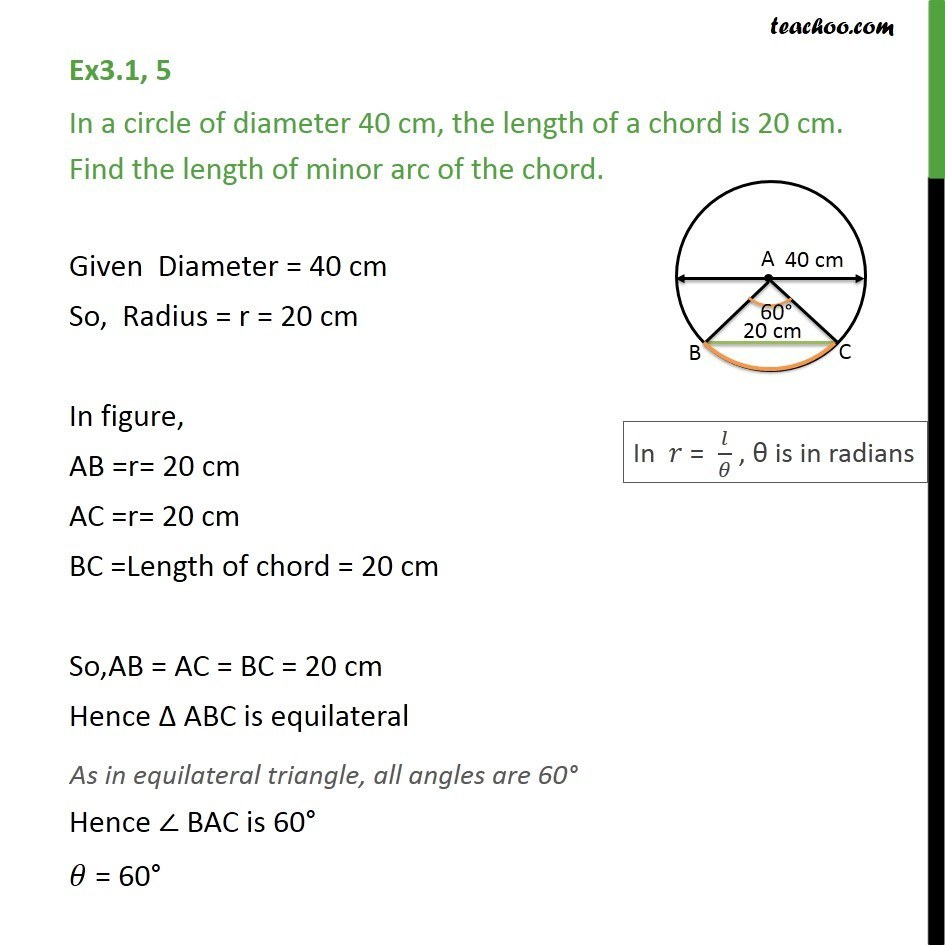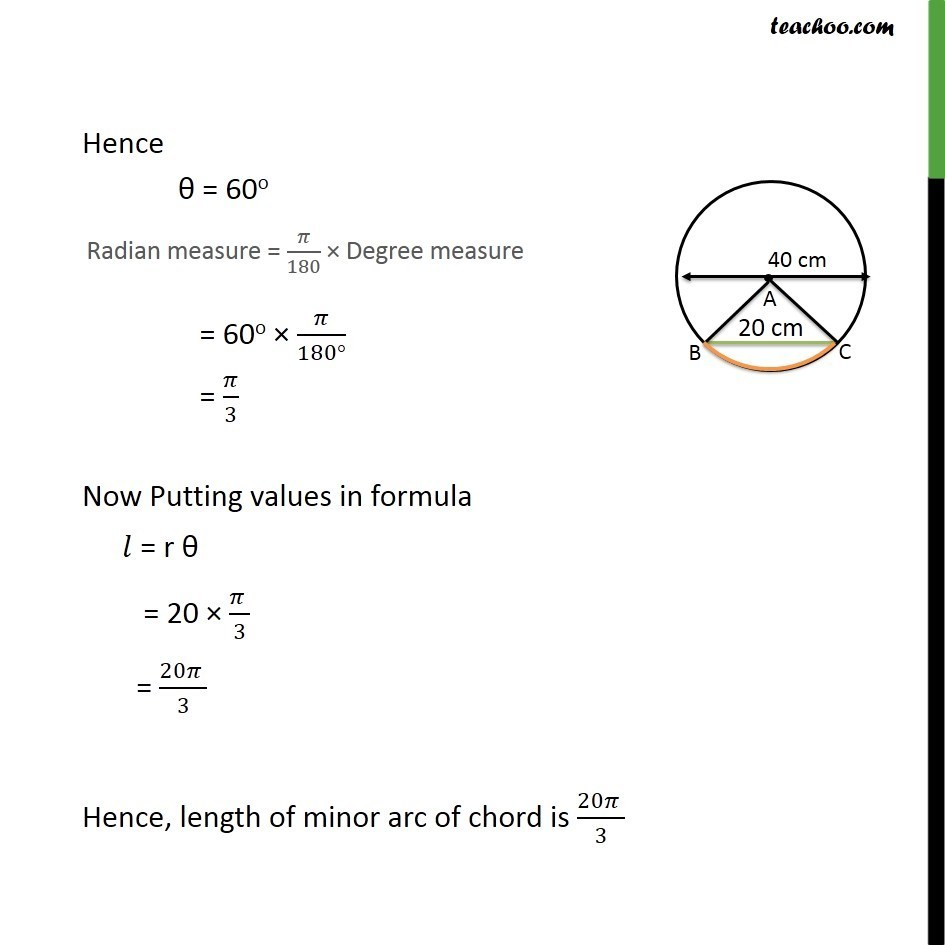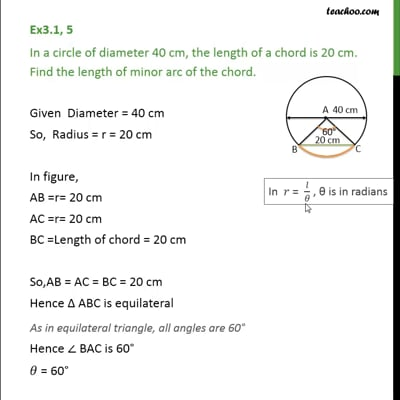Arc length

Chapter 3 Class 11 Trigonometric Functions
Concept wiseThis video is only available for Teachoo black users

Introducing your new favourite teacher - Teachoo Black, at only ₹83 per month

### Transcript

Ex3.1, 5 In a circle of diameter 40 cm, the length of a chord is 20 cm. Find the length of minor arc of the chord. Given Diameter = 40 cm So, Radius = r = 20 cm In figure, AB =r= 20 cm AC =r= 20 cm BC =Length of chord = 20 cm So,AB = AC = BC = 20 cm Hence ABC is equilateral Hence BAC is 60 = 60 Hence = 60o = 60o /(180 ) = /3 Now Putting values in formula = r = 20 ( )/3 = (20 )/3 Hence, length of minor arc of chord is (20 )/3